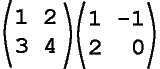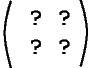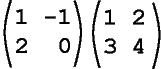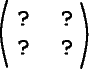Is it always true that AB = BA?

NO!   Even when both products can be formed they are rarely equal.

# Not Commutative

For example, calculate both of the following products (you might wish to use paper and pencil):

ProblemThink FirstResultThis shows that in general for matrices A and B,   ABBA.

### QUESTION 8:

Is A4×4 04×4  =  04×4 A4×4,   where 0 is the zero matrtix?